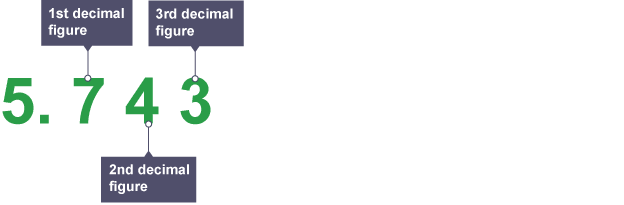# Maths Support Week 4 Summer 1

Next week the children will be focussing on rounding within their Maths. The children will round decimals with two decimal places to the nearest whole number and to one decimal place. They will also read, write, order and compare numbers with up to three decimal places.

# Rounding to a given number of places

## Counting decimal places

Decimal places are counted from the decimal point:So, the numberhas four decimal places, whilehas two decimal places.

To round a number to a given number of decimal places, look at the number in the next decimal place:

• If it’s less than, round down
• If it’sor more, round up

### Example

Roundtodecimal places

This means we needdigits after the decimal point.Because the next digit, is more than, we round theup.(decimal places).

## 2 thoughts on “Maths Support Week 4 Summer 1”

1.EmilyG

Hi Mr Carr I have done some rounding to decimal places my self here are some of them.

567.589 one decimal place=567.6 two decimal places=567.59
127.43 one decimal place=127.4 two decimal places=127.43
8.86 one decimal place=8.9 two decimal places=8.86
50.71 one decimal place=50.7 two decimal places=50.71

I hope I got these right now I really understand decimal places and rounding than before this comment is from E.G

1.l.carr Post author

Excellent work Emily. You’re more than ready to tackle the rounding problems next week!